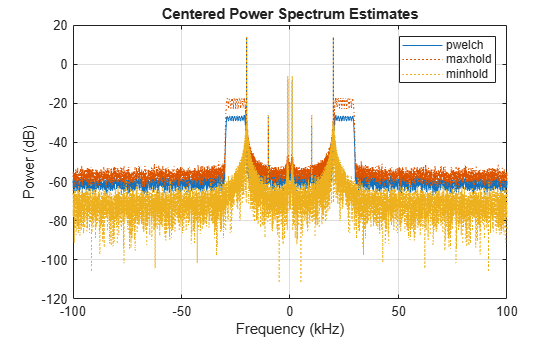# Welch Spectrum Estimates

Create a signal consisting of three noisy sinusoids and a chirp, sampled at 200 kHz for 0.1 second. The frequencies of the sinusoids are 1 kHz, 10 kHz, and 20 kHz. The sinusoids have different amplitudes and noise levels. The noiseless chirp has a frequency that starts at 20 kHz and increases linearly to 30 kHz during the sampling.

```Fs = 200e3; Fc = [1 10 20]'*1e3; Ns = 0.1*Fs; t = (0:Ns-1)/Fs; x = [1 1/10 10]*sin(2*pi*Fc*t)+[1/200 1/2000 1/20]*randn(3,Ns); x = x+chirp(t,20e3,t(end),30e3);```

Compute the Welch PSD estimate and the maximum-hold and minimum-hold spectra of the signal. Plot the results.

```[pxx,f] = pwelch(x,[],[],[],Fs); pmax = pwelch(x,[],[],[],Fs,'maxhold'); pmin = pwelch(x,[],[],[],Fs,'minhold'); plot(f/1000,pow2db(pxx)) hold on plot(f/1000,pow2db([pmax pmin]),':') hold off xlabel('Frequency (kHz)') ylabel('PSD (dB/Hz)') legend('pwelch','maxhold','minhold') grid```Repeat the procedure, this time computing centered power spectrum estimates.

```[pxx,f] = pwelch(x,[],[],[],Fs,'centered','power'); pmax = pwelch(x,[],[],[],Fs,'maxhold','centered','power'); pmin = pwelch(x,[],[],[],Fs,'minhold','centered','power'); plot(f/1000,pow2db(pxx)) hold on plot(f/1000,pow2db([pmax pmin]),':') hold off xlabel('Frequency (kHz)') ylabel('Power (dB)') legend('pwelch','maxhold','minhold') title('Centered Power Spectrum Estimates') grid```